# Nested Lattices of E8 in Complex Projective 4-Space

I read an interesting article about a pattern discovered by Warren D. Smith (discussed at length here):

“The sum of the first three terms in the Eisenstein E_4(q)  Series Integers of the Theta series of the E8 lattice is a perfect fourth power: 1 + 240 + 2160 = 2401 = 7^4”

So I decided to visualize the 2401=1+240+2160 vertex patterns of E8 using my Mathematica codebased toolset based on some previous work I put on my Wikipedia talk page.

The image below represents various projections showing 6720 edges of the 240 E8 vertices, plus a black vertex at the origin, and the 2160 Witting Polytope E8 2 _ 41 vertices using the same projection basis (listed at the top of each image along with the color coded vertex overlaps). Click these links for a higher resolution PNG or the SVG version.

Some of the particular projections of the Witting Polytope may need 8D rotations applied to the basis vectors to find better symmetries with the Gosset, but this is a start using my standard set of projections.

The 240 vertices of the Gosset Polytope are generated using various permutations:

(* E8 4_21 vertices *)
e8421 = Union@Join[
Eperms8@{1, 1, 1, 1, 1, 1, 1, 1}/2,
perms8@{1, 1, 0, 0, 0, 0, 0, 0}];

The 2160 vertices of the Witting Polytope are generated using various permutations:

(* E8 2_41 vertices *)
e8241=Union@Join[
perms8[{1,0,0,0,0,0,0,0}4],
perms8[{1,1,1,1,0,0,0,0}2],
Eperms8[({2,0,0,0,0,0,0,0}+1)]]/4;

Another view shows just the 2160 Witting Polytope vertices. Click these links for a higher resolution PNG or the SVG version.

Another great source of visualizations on E8 and this Witting Polytope is here.

Now visualizing in 3D the structure in 3D using rows 2-4 of the E8->H4 folding matrix, we get:Witting Polytope with 2160 E8 2 _ 41 vertices sorted by Norm distance into 3D Platonic solid related concentric hull structures using rows 2-4 of the E8->H4 folding matrix. The last image shows overlap counts using colored vertices.

# Visualizing the concentric hulls of E8 with the 24-cells of H4 & H4Φ

I’ve had a number of related posts on this topic, but I wanted to present a few new pictures and PDF documents that combine the 7 concentric hulls forming Platonic solid related sets of E8 vertices projected to 3D with vertices of five pairs of 24-cell objects.

These break down the E8 structure into familiar 3D objects, such as the icosahedron with its dual the dodecahedron, the icosidodecahdron, and the 16-cell with its dual the 8-cell (aka. Tesseract) combined to create the self-dual 24-cell.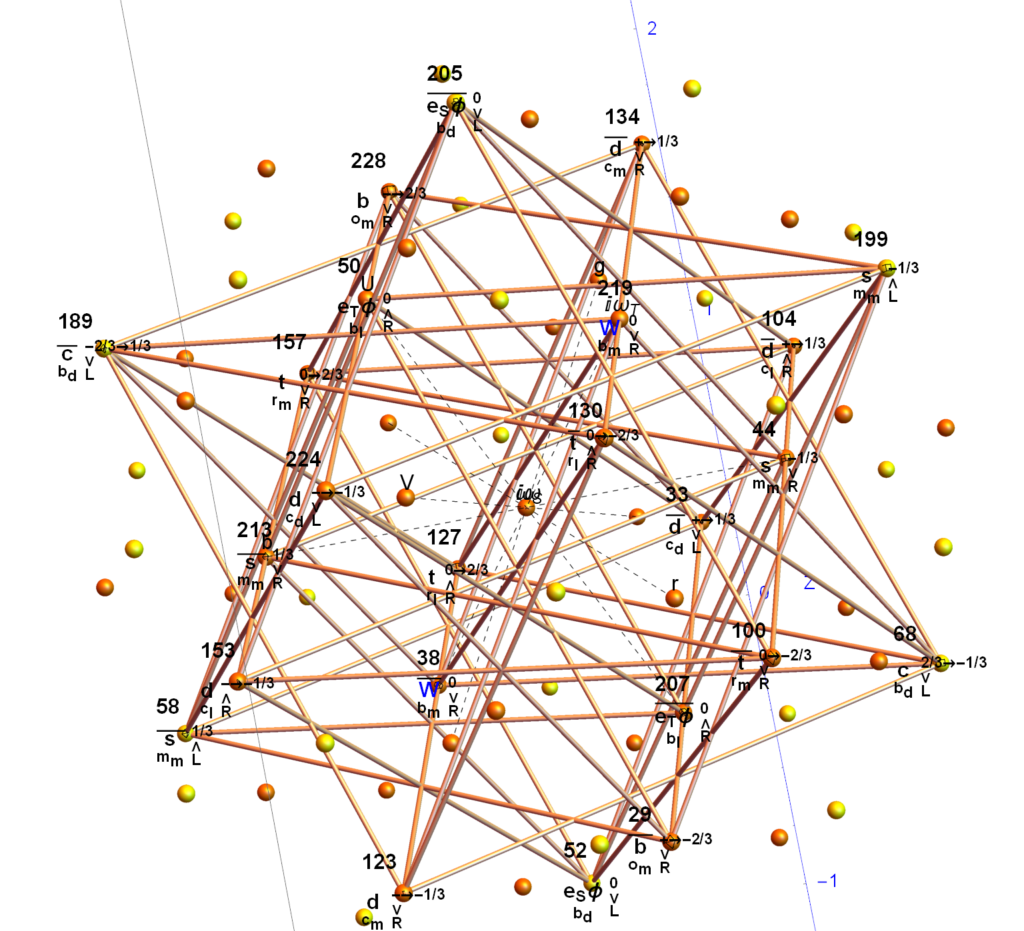The yellow spheres are those of the outer hull of singular 30 vertices of an icosidodecahedron. The orange spheres are pairs of icosahedron or dodecahedron vertices. The edges with numbered vertices form one of five 24-cells in the 120 vertex H4Φ scaled 600-cell within E8. Specifically, it is the 3rd rotation (of 4 π/5 rotations) within the 96 vertices of the snub-24-cell. You can see that 6 of the yellow vertices connect to the 24-cell. Rotating that 24-cell four times in 3-space by π/5 gives the connections to the rest of the vertices in H4Φ and completes the 30 vertex icosidodecahedron . The same is true for the 120 vertices of H4 using the corresponding 24-cell in H4.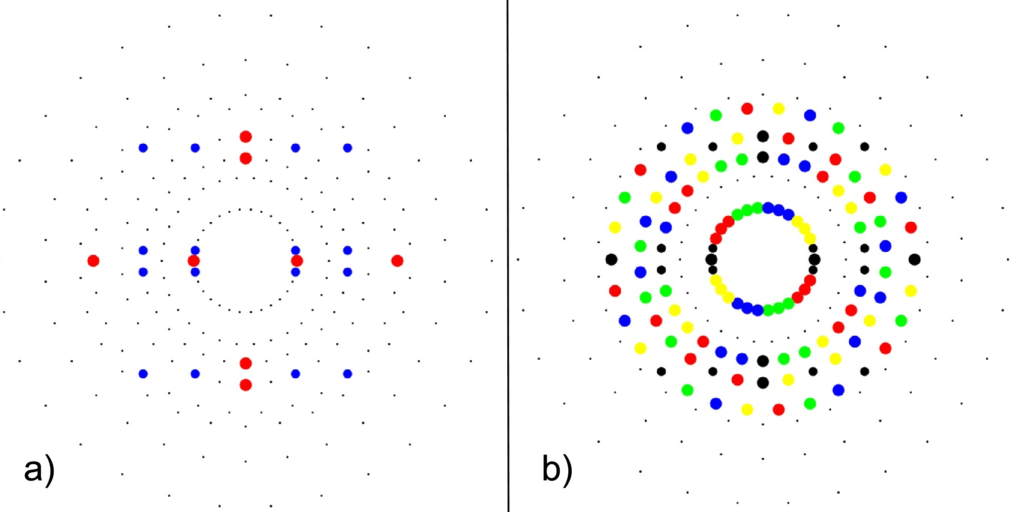a) 24-cell highlighting the 16-cell (red on-axis vertices) and 8-cell (blue off-axis vertices)b) Snub 24-cell highlighting four π/5 rotations of the 24-cell (black) in red, green, blue, yellow.

The following paper hulls-24cells-combined.pdf (13MB) & interactive Mathematica Notebook hulls-24cells-combined.nb (34MB) contains a comprehensive set of images that show the contents of the 7 concentric hulls of Platonic solid related shapes as well as their integration with one of the rotations of 24-cells in H4 and H4Φ in E8 (the same one used in the image above).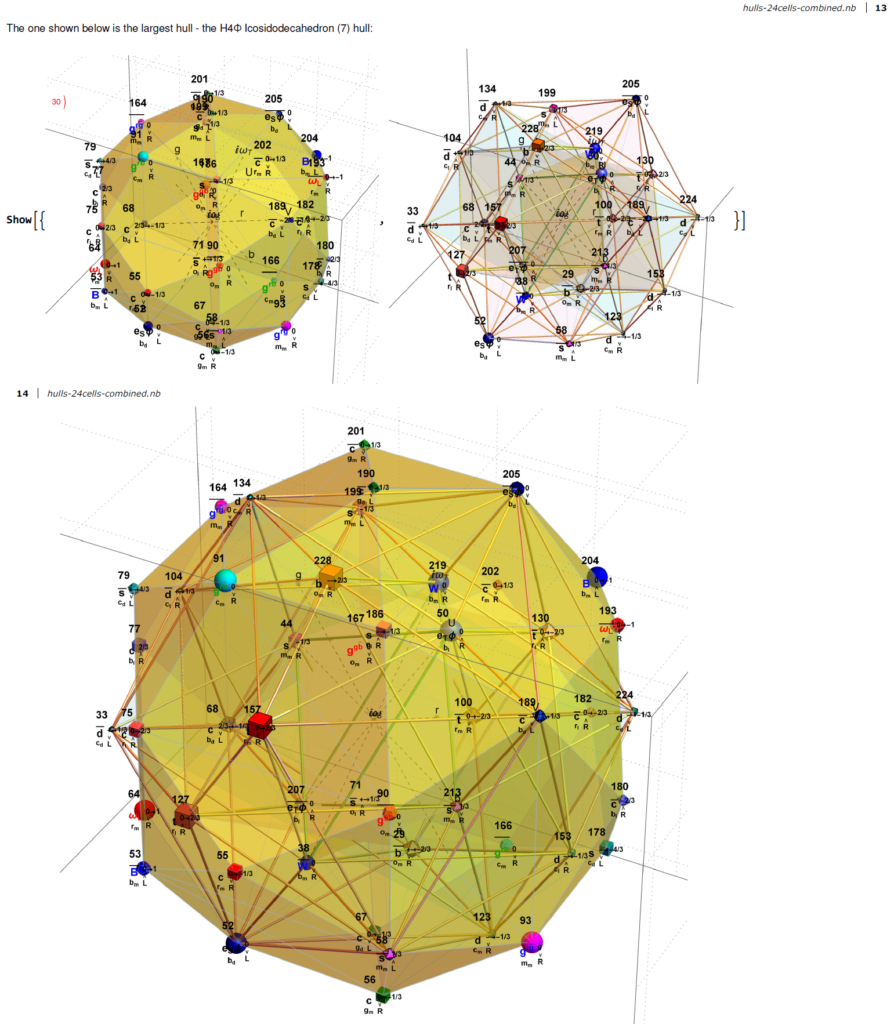Snapshot from the paper showing the 3rd rotation of the snub 24-cell and the icosidodecahedron of H4Φ

In the E8 Petrie projection, every hull (each with 2 or 4 overlapping vertices) pair into left/right patterns. See the set of four icosahedrons that occupy the 3rd hull in both 2D and 3D (the yellow edge sets belongs to the H4Φ and the blue sets belong to H4) :

The pair of dodecahedrons that occupy the 5th hull in both 2D and 3D (the yellow edge set belongs to the H4Φ and the blue belong to H4) :

Below is an animation that cycles through the sequence of 2D Petrie Projections of the pairs of hulls. One cycle shows each frame individually and the other builds the E8 Petrie from the previous 2D hull.

Below are images of the left (H4) and right (H4Φ) 2D Petrie projections of the hulls (which are defined by the Norm’s of 3D projected vertices using the E8->H4 folding matrix rows 2-4 as basis vectors). The two 30 vertex 4 & 7 Icosidodecahedron hulls and four 0th hull (points) are omitted leaving the gaps in the diagram. When these gap vertices are combined with the 48 3rd hull Icosahedrons (above), they make up the 112 integer D8 group assigned to the Bosons (48) and 2nd generation Fermions (64) in the physics model described below.

The combined set of hulls projected using the rows of the folding matrix as basis vectors is shown below from previous work:

This paper hull-list-3b.pdf (4MB) & interactive Mathematica Notebook hull-list-3b.nb (20MB) contains the visualizations and detail E8 vertices associated with each Platonic solid related concentric hulls. This paper 24cell-list-3b.pdf (6MB) & interactive Mathematica Notebook 24cell-list-3b.nb (20MB) contains the visualizations and detail for the five 24-cells in each H4 & H4Φ. Both of these papers also have detail information about its assigned physics particle based on a modified A.G. Lisi model (shown below). This paper describes these particle assignment symmetries in more detail.

# Latest Paper – Unimodular rotation of E8 to H4 600-cells

## Please see my latest paper that describes some advances in understanding the E8 to H4 rotation matrix

https://theoryofeverything.org/TOE/JGM/Unimodular-Rotation-of-E8-to-H4.pdf

Abstract: We introduce a unimodular Determinant=1 8×8 rotation matrix to produce four 4 dimensional copies of H4 600-cells from the 240 vertices of the Split Real Even E8 Lie group. Unimodularity in the rotation matrix provides for the preservation of the 8 dimensional volume after rotation, which is useful in the application of the matrix in various fields, from theoretical particle physics to 3D visualization algorithm optimization.

# Stereoscopic 3D Interactive Solar system simulation (shoemaker-Levy-9)

## It requires local installation of the free Mathematica CDF plugin.

This now includes the 1998 OR2 “Planet Killer” that will pass very near Earth in April 2020 (when selecting the Asteroid vs. Comet).

Now with improved UI, better planet scaling, and Anaglyph viz.

# Mathematica Analysis of Cohl Furey’s octonion and Clifford group theoretic ℂ⊗O assignments to standard model particles

I’ve read some recent papers by Cohl Furey and was intrigued by the potential relationship between the octonion and Clifford group theoretic assignments to standard model particles. Since I have developed an extensive Mathematica notebook to perform symbolic analysis using these structures (derived primarily for E8 Lie group work), I decided to follow her suggestion… “The reader is encouraged to check that C⊗O forms the 64-complex-dimensional Clifford algebra Cl(6), generated by the set {i e1,…i e6} acting on f”.

So to that end, I created the this .pdf with some preliminary results of that analysis. I found a few minor issues, but so far the model seems to symbolically compute consistently. Very cool!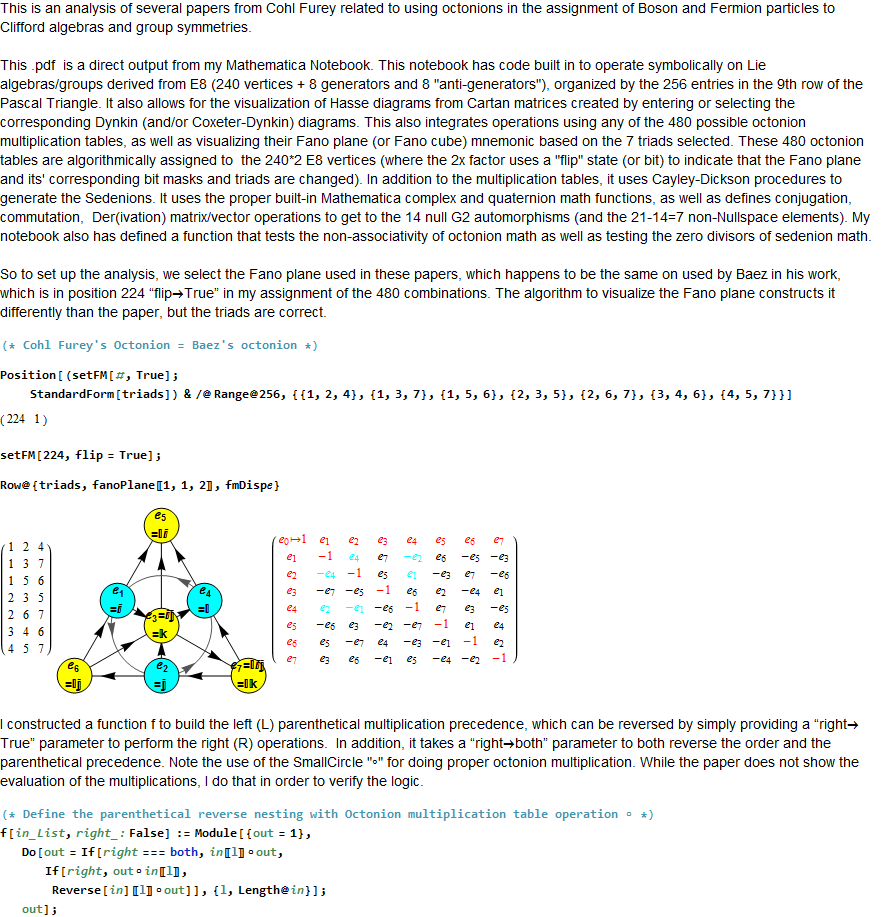# Latest paper: Even FibBinary Numbers and the Golden Ratio

Please see my latest paper here.

It adds information related to my previous blog post here.

Abstract: Previously, a determination of the relationship between the Natural numbers (N) and the n’th odd fibbinary number has been made using a relationship with the Golden ratio phi=(Sqrt+1}/2 and tau=1/phi. Specifically, if the n’th odd fibbinary equates to the j’th N, then j=Floor[n phi^2 – 1]. This note documents the completion of the relationship for the even fibbinary numbers, such that if the n’th even fibbinary equates to the j’th N, then j=Floor[n phi]-1, starting at j=0 for n=1. Alternatively, starting at j=2 for n=1, then j=Floor[n phi + tau].

Title:Even FibBinary Numbers and the Golden Ratio
Authors: J Gregory Moxness
Comments: Dec 31 2018, 5 pages, 4 Figures.
Subjects: Combinatorics

Below is a listing of the N integers represented by their 38 odd FibBinaries, along with the binary and Gray code Compositions.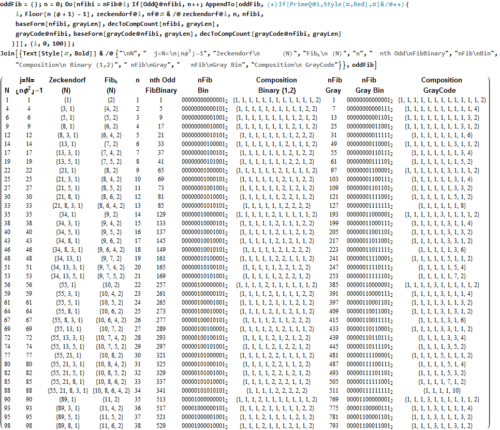Below is a listing of the first 63 N integers represented by their even FibBinaries, along with the binary and Gray code Compositions.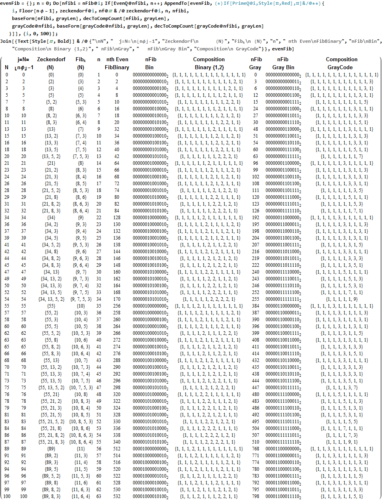Code output examples:Mathematica Code Snippets: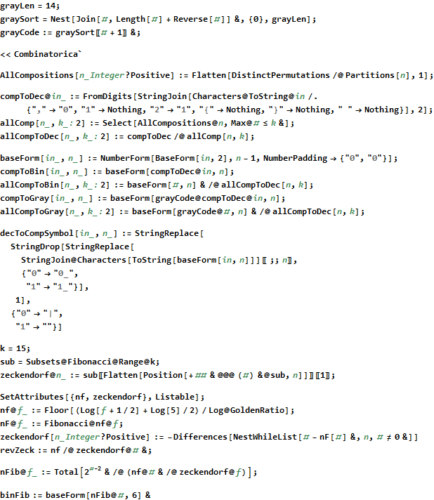# Integer Compositions, Gray Code , and the Fibonacci Sequence

I was prompted by Chris Birke to take a look at this very interesting paper by Linus Lindroos. Chris is working with my E8 to H4 Folding Matrix for optimizing computation using network theory and hyper-dimensional geometric structures.

I was able to validate the paper with some quick Mathematica code.

Below is the code that sets up the generation of the tables, including various binary, numeric, and symbolic (graph) Compositions.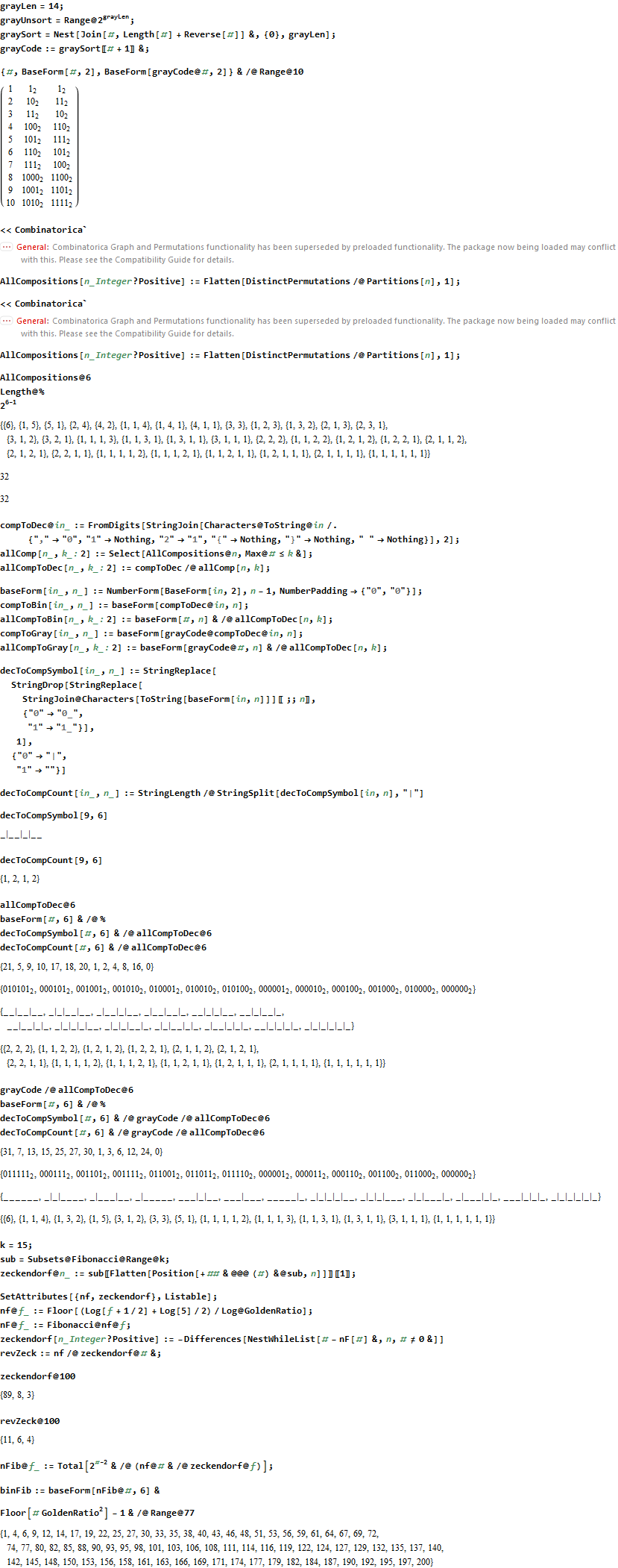Notice the last statement (above) lists the odd FibBinaries (up to N=200) as related to the Floor of the n*Phi^2-1, with n=1 to 77.

Below is a listing of the first 100 N integers represented by their odd and even FibBinaries, along with the binary and Gray code Compositions.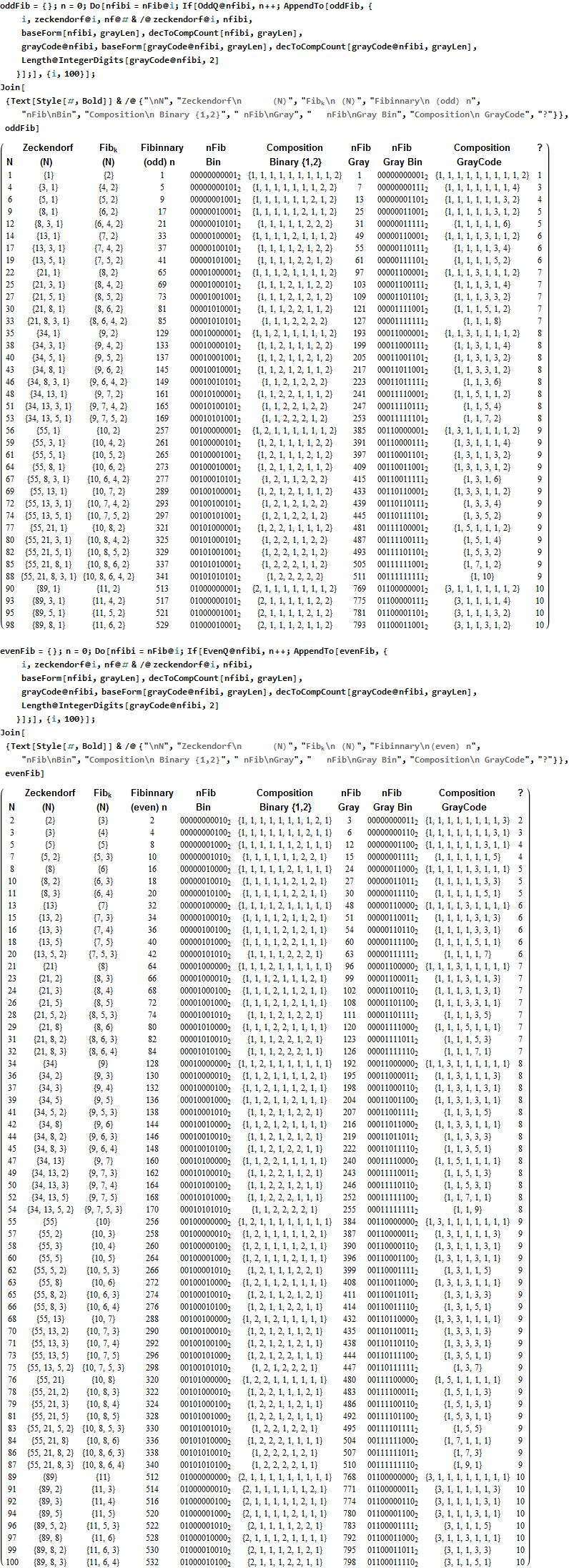# Working with E8+++

I wanted to confirm some work being done with E11 (or more specifically the Extended E8+++), so I used my “VisibLie” notebook (which includes the “SuperLie” package for analyzing Lie Algebras) to get the following information:

I first created the Dynkin diagram which produces the Cartan Matrix: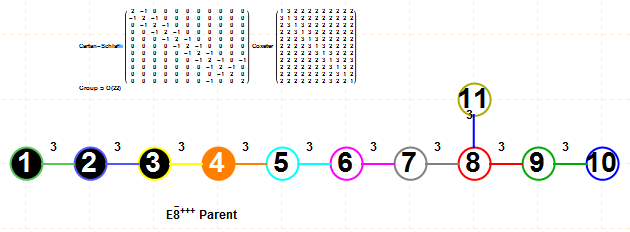This allows the evaluation of the EigenSystem of the Cartan matrix as follows: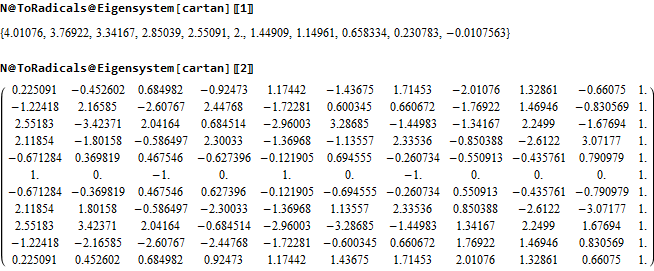As well as using the SuperLie package to evaluate the Positive Roots, Weights and Heights of the E8+++ (The first 80 are shown. For the full list in .pdf click here or click on the image below):The 4870 positive roots up to height 47 generate a Hasse diagram as follows (with 3 sections zoomed in):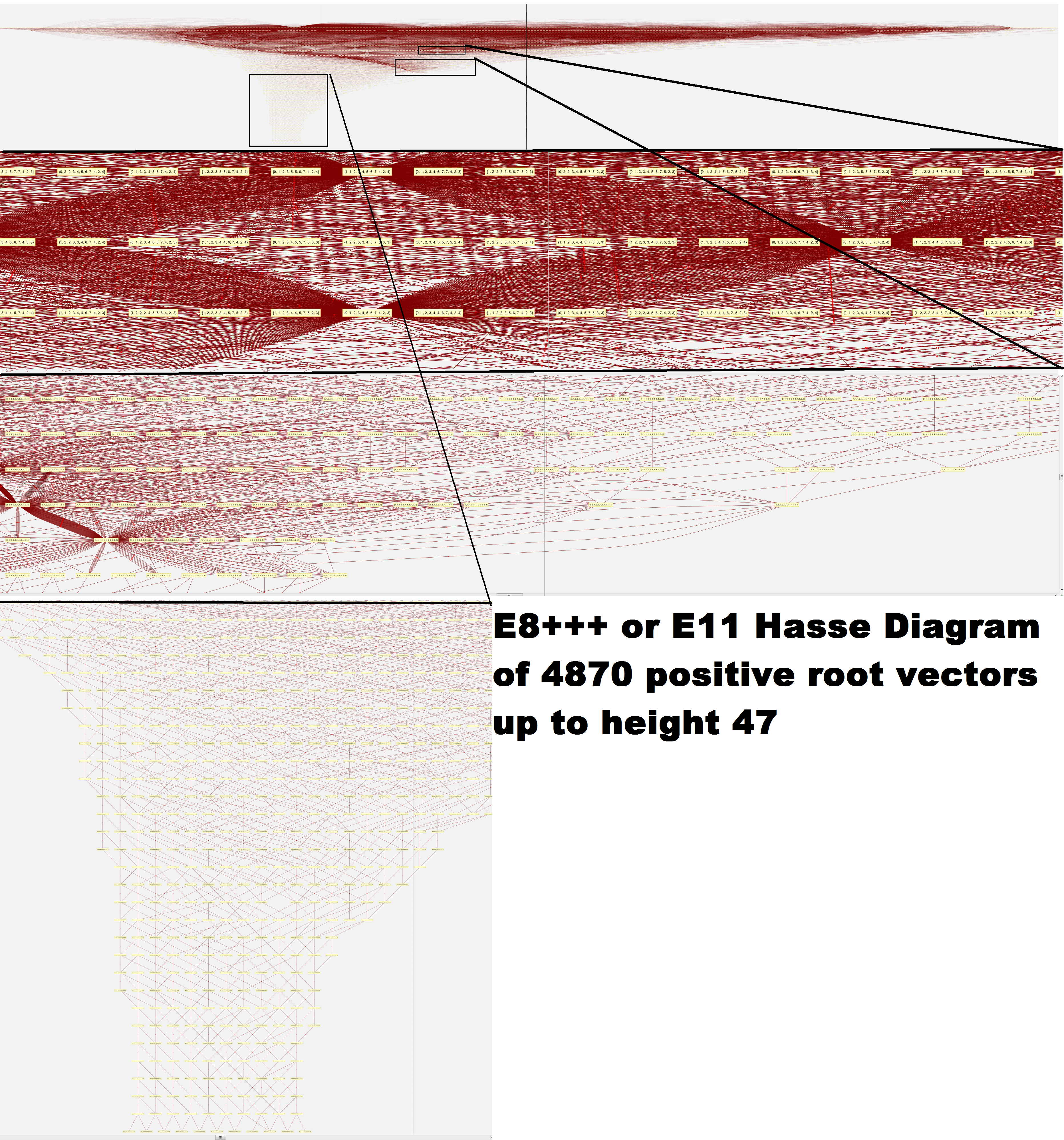I am working on replication of the Petrie projection by A.G. Lisi based on his prescription using his basis vectors for 2D projection and a Simple Roots Matrix: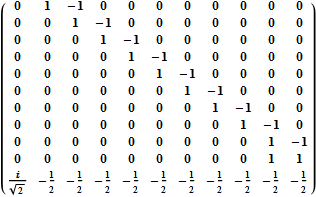Adjusting this E11 Simple Roots Matrix (srmE11) to match the Dynkin diagram above and then verifying it creates the same Cartan matrix, we have: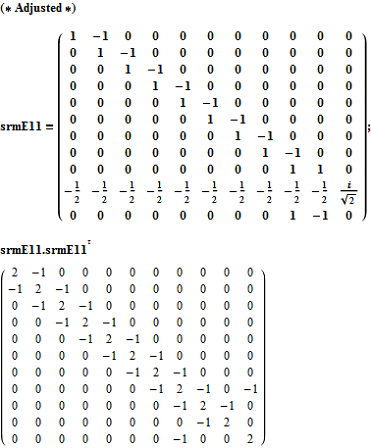To get the 9740 E8+++ vertices, we simply use the dot product of the transpose of srmE11 against the positive and negative root vectors (first 50 shown):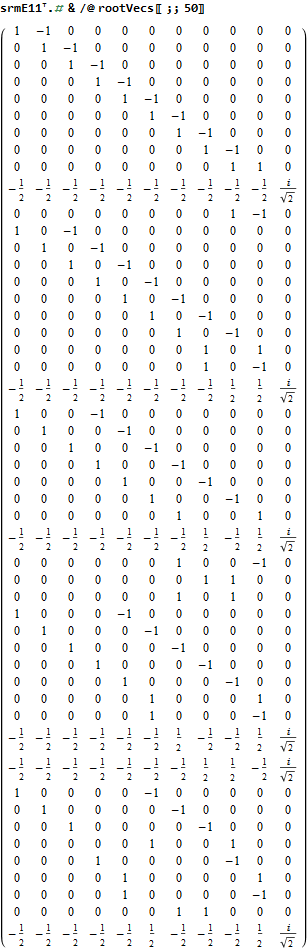A first cut at plotting these involves projecting them to a 2D using the dot product of the 2 basis vectors provided, which gives the following ListPlot: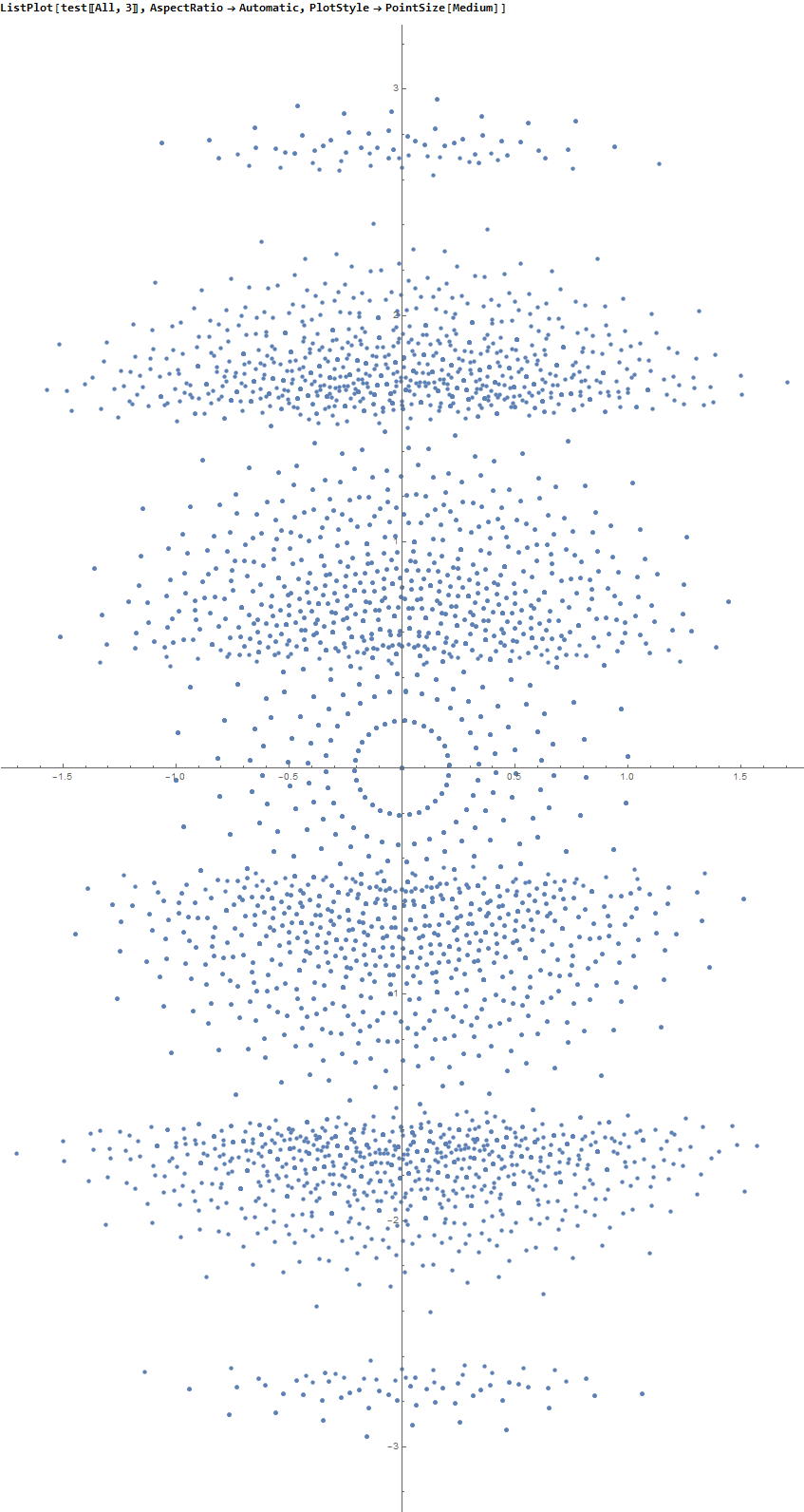The limited results from SuperLie are consistent with the image given by Lisi on the Bee’s BackReaction blog, after eliminating the vertical “levels” in my raw plot.

Email me if you want more detail, see errors, or would like to help.

Greg
JGMoxness@theoryofeverything.org